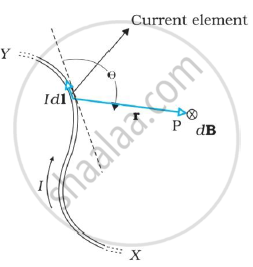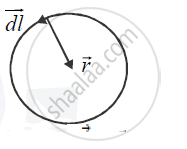Advertisement Remove all ads

# State and Explain the Law Used to Determine Magnetic Field at a Point Due to a Current Element. Derive the Expression for the Magnetic Field Due to a Circular Current Carrying Loop of Radius R - Physics

Answer in Brief

State and explain the law used to determine magnetic field at a point due to a current element. Derive  the expression for the magnetic field due to a circular current carrying loop of radius r at its centre.

Advertisement Remove all ads

#### Solution

The law which gives the magnetic field at a point due to a current  element, is Biot-Savart Law.
It states that,if we consider an infinitesimal element dl of the
conductor,the magnetic field dB due to this
element can be determined at a point P at a distance r from it, as follows:
Let θ be the angle between dl and the displacement vector r.
According to Biot-Savart’s law,the magnitude of the magnetic field dB is proportional tothe current I, the element length |dl|,and inversely proportional to the square of the distance r. Its direction is  perpendicular to the plane containing dl and r .Thus, in vector notation, d vec( B )  α  I (d vec (l)xx vec(r))/(r^3)

where μ0 / 4π is a constant of proportionality. The above expression holds when the medium is vacuum.The magnitude of this field is,

|d vec(B) | = (mu_0)/(4pi) (Idlsin theta )/(r^2)

The proportionality constant in SI units has the exact value,

(mu_0)/(4pi) = 10^(-7) T - m/A

We call μ0 the permeability of free space (or vacuum).

Magnetic field at the centre of a circular loop of radius r carrying current I:

We consider a small element of length dl. The vec (r) joining the centre of the circle to this element is  perpendicular tod vec(l) . Hence the magnitude of magnetic field at the centre due to  this element (by Biot-Savart Law) is

dB = (mu_0)/(4 pi) (Idl sin theta)/(r^2) or dB=  (mu_0)/(4 pi) (Idl sin 90^circ)/(r^2)  = (mu_0)/(4pi) (Idl)/(r^2)This field is perpendicular to the plane of the coil (as it is directed along dvec(l) xx vec (r)).
Hence, net field due to the entire circular loop, is

B = int  (mu_0)/(4 pi) (Idl )/(r^2) = (mu_0)/(4 pi) (I)/(r^2) int dl = (mu_0)/(4 pi) xx(I)/(r^2) xx 2 pir

Or B = (mu_0I)/(2r)

Is there an error in this question or solution?
Advertisement Remove all ads

#### APPEARS IN

Advertisement Remove all ads

#### Video TutorialsVIEW ALL 

Advertisement Remove all ads
Share
Notifications

View all notifications

Forgot password?